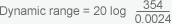Home/Resources/Ask The Experts/Understanding IEPE Accelerometer Dynamic Range

# Understanding IEPE Accelerometer Dynamic Range

### Question

Question: Please explain the concept of dynamic range, as it applies to an IEPE accelerometer? I cannot seem to find this information on Endevco® product data sheets.

IEPE accelerometer dynamic range can be defined as the range between lowest and highest levels that an accelerometer can measure. Accelerometer dynamic range can be defined in many ways; this article, however, will cover the most commonly used method for estimating transducer dynamic range. When making this estimation, we generally refer to a term known as residual noise, or resolution, to determine our minimum discernible signal and our maximum amplitude range. As dynamic range is expressed in terms of decibels (dB), the following equation is used to make this type of calculation:

• Dynamic Range = 20 log (range/mds)

Where:

• range = specified range in g's (RMS)

• mds = minimum discernible signal, which is the residual noise x 3

As an example, let's take an Endevco® model 65-10. This is an IEPE (offered by Endevco as ISOTRON®) triaxial accelerometer with a 10 mV/g sensitivity and the following specifications:

• Range: ±500 g peak

• 500 X 0.707 = 354 g RMS

• Resolution: 0.0008 g RMS (800 µg)

• Bandwidth: 2 Hz to 10 kHzThus, the dynamic range of the Endevco® model 65-10 is 103.4 dB.

So, in this calculation, why did we multiply the residual noise level times three? This is because we are trying to determine a level where it is possible to see actual signal. If we simply used the noise level specification, we assume that if the signal level equals the noise level, we could see the signal, a concept or assumption which is incorrect.

In the above example, the range was converted to RMS, so that the terms are consistent.

In practice, when determining the minimum discernible signal, it is necessary to take into account the noise contribution of any additional amplification in the string. Noise improvement can also be realized by filtering out any out-of-band noise.